11月销量破7000，哈弗F5“爆款”潜质显露无遗

无论市场大环境如何变迁，市场的基本规律不会改变，满足消费者需求的好产品，即使在整体不景气的大环境下，依旧能够逆势上扬，斩获出众业绩。哈弗F5就是其中的典型。继续上市首月成功斩获6265台的月销成绩后，11月哈弗F5延续了首月的热销势头，单月销量达7065辆，环比增长12.77%。面对国内强者如林的紧凑SUV市场，横空出世的哈弗F5靠什么站稳脚跟，并不断创造新的可能？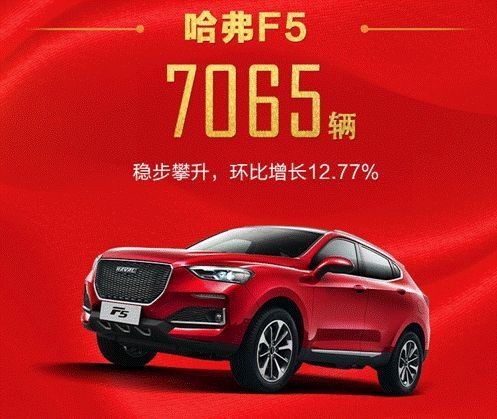以“用户思维”打造新潮爆款

当下的90后年轻人早已不相信任何预设的标签，靠一个车标无限“拉皮”就能赚钱的老套路在他们这儿早已彻底失效。只有在产品层面摸准年轻人的用车喜好和习惯，为年轻人量身打造专属座驾，才能真正获得年轻消费者的认可。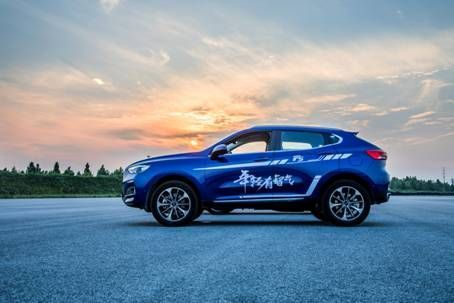在外观方面，采用“尖锋Battle”设计美学的哈弗F5，将年轻化的姿态贯穿全车。大面积的点阵式进气格栅，层次分明、动感十足的车侧轮廓，简洁饱满的尾部造型。从整体气场，到可局部特征，无不展现出哈弗F5的潮派、时尚与动感气息，完美地符合了年轻消费者的个性期待。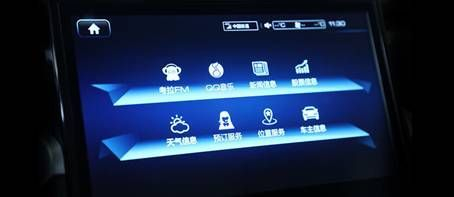哈弗紧贴年轻人无界互联的出行需求，而哈弗F5搭载了哈弗互联3.0系统，2000万高品质在线音乐+10万档在线电台娱乐节目，从美国billboard公告牌榜，到英国UK榜，再到香港电台榜等全球榜单悉数囊括；高达97%的语音识别率，让年轻车主在谈笑之间就可以随时了解的时事新闻、天气信息。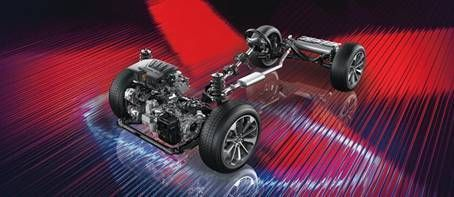此外，哈弗F5不仅对动力系统进行了数据优化，更对其底盘进行了专业调校，令哈弗F5在过弯时支撑性更强、更加运动化，其所搭载的1.5T发动机，拥有125kW、285N·m超强动力，为年轻人带来了他们热爱的驾驶乐趣。

用年轻姿态释放新潮魅力

作为哈弗品牌年轻化的排头兵，哈弗F5试图征服年轻消费群体的努力，除了在产品层面得到了淋漓尽致的体现，在营销玩法上，同样紧贴年轻人群，不断释放新潮魅力。

相对于传统车企以稳为主的营销套路，哈弗F5自诞生之初便选择了更符合年轻消费人群沟通语境的“走位”。为了让产品形象的高效覆盖、传达在精准人群的聚集地，哈弗F5选择了与拥有优质流量和爆款基因的年轻人聚集地——抖音APP合作，开启汽车行业在抖音“对战”超级挑战赛的首秀。截至10月初，挑战赛带来了强效互动和曝光价值，24万用户共计上传36.5万支视频，收获6000万+点赞，话题总曝光量超23.2亿，成就汽车行业玩法新标杆。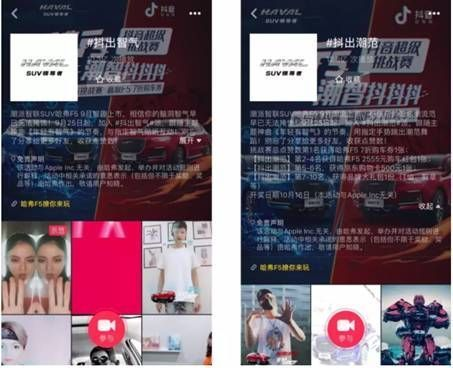同时，上市之初，哈弗F5还携手年轻消费者热衷的综艺《蒙面唱将》进行跨界营销，借助娱乐综艺的强大影响力，潜移默化中将哈弗F5的年轻化形象传递给广大消费者。

此外，哈弗为年轻人推出了诚意满满的优惠政策，即日起到12月31日，购买哈弗F5享受购置税减半优惠！活动期间，凡购买哈弗F5的消费者，均可在新车指导价的基础上享受50%的购置税补贴。以一辆官方指导价为12.8万元的哈弗F5 i范为例，折算下来，消费者可享受高达5470元的实惠！

用年轻人热爱的方式，和年轻人打成了一片，哈弗F5创下了车企以年轻用户思维出发的全新营销模式，紧扣年轻人喜好的跨界合作、新潮营销，使哈弗品牌和年轻目标消费群体中实现了深入的良性互动。

哈弗F5的持续热销，是哈弗F系顺利顺利开局的一小步，更是哈弗品牌年轻化的一大步。随着哈弗F7的上市和开局热销，哈弗F系已然正式成军。可以预见，在哈弗F系的有力驱动下，一个更加年轻、更加智能的哈弗品牌，正在加速向我们驶来。

##### 相关资讯

|分享

•已阅！握爪
•我手滑为你点赞
•128个赞！
•不明觉厉
•阅后既醉
•有钱！任性
•照片太美，我不敢看
•干货！杠杠的
•高大上
•膜拜中！
•请接受我的膝盖
•猫哥/猫妹么么哒
•神吐槽
•我只笑笑不说话
•我想静静
•窒息
##### 资讯相关车系##### 热度排行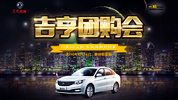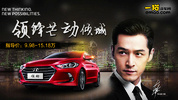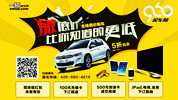• 阿斯顿·马丁
• 奥迪
• 阿尔法罗密欧
• ALPINA
• 爱驰
• AC Schnitzer

• 巴博斯
• 宝骏
• 宝马
• 保时捷
• 北汽制造
• 奔驰
• 奔腾
• 本田
• 比亚迪
• 标致
• 别克
• 宾利
• 布加迪
• 北汽威旺
• 北京
• 北汽绅宝
• 北汽幻速
• 北汽新能源
• 宝沃
• 比速汽车
• 北汽道达
• 北京汽车
• 比德文汽车
• 铂驰
• 北汽昌河

• 昌河
• 长安
• 长城
• 长安商用
• 成功汽车
• 长江EV
• 长安轻车型
• 长安欧尚
• 长安凯程
• 长安跨越
• 车驰汽车

• 大众
• 道奇
• 东风
• 东风风神
• 东风小康
• 东南
• DS
• 东风风行
• 东风风度
• 东风风光
• 电咖
• 大乘汽车
• 大运
• 东风富康

• 法拉利
• 菲亚特
• 丰田
• 福迪
• 福特
• 福田汽车
• 福汽启腾
• 风诺
• 福田

• GMC
• 广汽传祺
• 广汽吉奥
• 观致
• 国金汽车
• 广汽新能源
• 广汽集团
• 国机智骏

• 哈飞
• 海格
• 海马
• 华泰
• 黄海
• 恒天
• 红旗
• 哈弗
• 华颂
• 华凯
• 华泰新能源
• 汉腾汽车
• 华利
• 红星汽车
• HYCAN合创
• 汉龙汽车

• Jeep
• 江淮
• 江铃
• 捷豹
• 金杯
• 九龙
• 吉利汽车
• 金旅
• 金龙
• 江铃集团轻汽
• 江铃集团新能源
• 君马汽车
• 捷途
• 钧天
• 捷达
• 几何汽车

• 开瑞
• 凯迪拉克
• 科尼赛克
• 克莱斯勒
• KTM
• 卡威
• 凯翼
• 康迪
• 康迪电动汽车集团
• 开沃汽车
• 卡升

• 猎豹汽车
• 兰博基尼
• 劳斯莱斯
• 雷克萨斯
• 雷诺
• 理念
• 力帆
• 莲花汽车
• 林肯
• 铃木
• 陆风
• 路虎
• 路特斯
• 领志
• 领克
• 零跑汽车
• Lorinser
• LOCAL MOTORS
• LITE
• 领途汽车
• 理想汽车
• 罗夫哈特
• 力帆汽车

• MG
• MINI
• 马自达
• 玛莎拉蒂
• 迈凯伦
• 摩根
• 名爵
• 迈莎锐
• 迈迈

• 纳智捷
• 哪吒汽车

• 讴歌
• 欧宝
• 欧朗
• 欧拉
• 欧尚汽车

• 帕加尼
• Polestar极星

• 奇瑞
• 启辰
• 起亚
• 前途
• 庆铃汽车
• 骐铃汽车
• 全球鹰
• 乔治·巴顿

• 日产
• 荣威
• 瑞麒汽车
• 如虎
• 瑞驰
• 瑞麒
• 瑞驰新能源
• 容大智造

• 上汽大通
• smart
• 三菱
• 双环
• 双龙
• 斯巴鲁
• 斯柯达
• 萨博
• 思铭
• 赛麟
• SWM斯威汽车
• 上汽MAXUS
• SRM鑫源
• 思皓
• SHELBY
• 上喆

• TESLA
• 泰卡特
• 腾势
• 特斯拉
• 天际汽车

• 威麟
• 威兹曼
• 沃尔沃
• 五菱汽车
• 五十铃
• 潍柴英致
• WEY
• 蔚来
• 威马汽车
• 潍柴汽车

• 现代
• 雪佛兰
• 雪铁龙
• 西雅特
• 新特汽车
• 小鹏汽车
• 新宝骏

• 野马汽车
• 一汽
• 依维柯
• 英菲尼迪
• 永源
• 驭胜
• 云度
• 宇通客车
• 云雀汽车
• 远程汽车
• 一汽凌河

• 中华
• 中兴
• 众泰
• 知豆
• 之诺
• 正道汽车
• A
• B
• C
• D
• E
• F
• G
• H
• I
• J
• K
• L
• M
• N
• O
• P
• Q
• R
• S
• T
• U
• V
• W
• X
• Y
• Z

• 阿斯顿·马丁
• 奥迪
• 阿尔法罗密欧
• ALPINA
• 爱驰
• AC Schnitzer

• 巴博斯
• 宝骏
• 宝马
• 保时捷
• 北汽制造
• 奔驰
• 奔腾
• 本田
• 比亚迪
• 标致
• 别克
• 宾利
• 布加迪
• 北汽威旺
• 北京
• 北汽绅宝
• 北汽幻速
• 北汽新能源
• 宝沃
• 比速汽车
• 北汽道达
• 北京汽车
• 比德文汽车
• 铂驰
• 北汽昌河

• 昌河
• 长安
• 长城
• 长安商用
• 成功汽车
• 长江EV
• 长安轻车型
• 长安欧尚
• 长安凯程
• 长安跨越
• 车驰汽车

• 大众
• 道奇
• 东风
• 东风风神
• 东风小康
• 东南
• DS
• 东风风行
• 东风风度
• 东风风光
• 电咖
• 大乘汽车
• 大运
• 东风富康

• 法拉利
• 菲亚特
• 丰田
• 福迪
• 福特
• 福田汽车
• 福汽启腾
• 风诺
• 福田

• GMC
• 广汽传祺
• 广汽吉奥
• 观致
• 国金汽车
• 广汽新能源
• 广汽集团
• 国机智骏

• 哈飞
• 海格
• 海马
• 华泰
• 黄海
• 恒天
• 红旗
• 哈弗
• 华颂
• 华凯
• 华泰新能源
• 汉腾汽车
• 华利
• 红星汽车
• HYCAN合创
• 汉龙汽车

• Jeep
• 江淮
• 江铃
• 捷豹
• 金杯
• 九龙
• 吉利汽车
• 金旅
• 金龙
• 江铃集团轻汽
• 江铃集团新能源
• 君马汽车
• 捷途
• 钧天
• 捷达
• 几何汽车

• 开瑞
• 凯迪拉克
• 科尼赛克
• 克莱斯勒
• KTM
• 卡威
• 凯翼
• 康迪
• 康迪电动汽车集团
• 开沃汽车
• 卡升

• 猎豹汽车
• 兰博基尼
• 劳斯莱斯
• 雷克萨斯
• 雷诺
• 理念
• 力帆
• 莲花汽车
• 林肯
• 铃木
• 陆风
• 路虎
• 路特斯
• 领志
• 领克
• 零跑汽车
• Lorinser
• LOCAL MOTORS
• LITE
• 领途汽车
• 理想汽车
• 罗夫哈特
• 力帆汽车

• MG
• MINI
• 马自达
• 玛莎拉蒂
• 迈凯伦
• 摩根
• 名爵
• 迈莎锐
• 迈迈

• 纳智捷
• 哪吒汽车

• 讴歌
• 欧宝
• 欧朗
• 欧拉
• 欧尚汽车

• 帕加尼
• Polestar极星

• 奇瑞
• 启辰
• 起亚
• 前途
• 庆铃汽车
• 骐铃汽车
• 全球鹰
• 乔治·巴顿

• 日产
• 荣威
• 瑞麒汽车
• 如虎
• 瑞驰
• 瑞麒
• 瑞驰新能源
• 容大智造

• 上汽大通
• smart
• 三菱
• 双环
• 双龙
• 斯巴鲁
• 斯柯达
• 萨博
• 思铭
• 赛麟
• SWM斯威汽车
• 上汽MAXUS
• SRM鑫源
• 思皓
• SHELBY
• 上喆

• TESLA
• 泰卡特
• 腾势
• 特斯拉
• 天际汽车

• 威麟
• 威兹曼
• 沃尔沃
• 五菱汽车
• 五十铃
• 潍柴英致
• WEY
• 蔚来
• 威马汽车
• 潍柴汽车

• 现代
• 雪佛兰
• 雪铁龙
• 西雅特
• 新特汽车
• 小鹏汽车
• 新宝骏

• 野马汽车
• 一汽
• 依维柯
• 英菲尼迪
• 永源
• 驭胜
• 云度
• 宇通客车
• 云雀汽车
• 远程汽车
• 一汽凌河

• 中华
• 中兴
• 众泰
• 知豆
• 之诺
• 正道汽车
• A
• B
• C
• D
• E
• F
• G
• H
• I
• J
• K
• L
• M
• N
• O
• P
• Q
• R
• S
• T
• U
• V
• W
• X
• Y
• Z

• 阿斯顿·马丁
• 奥迪
• 阿尔法罗密欧
• ALPINA
• 爱驰
• AC Schnitzer

• 巴博斯
• 宝骏
• 宝马
• 保时捷
• 北汽制造
• 奔驰
• 奔腾
• 本田
• 比亚迪
• 标致
• 别克
• 宾利
• 布加迪
• 北汽威旺
• 北京
• 北汽绅宝
• 北汽幻速
• 北汽新能源
• 宝沃
• 比速汽车
• 北汽道达
• 北京汽车
• 比德文汽车
• 铂驰
• 北汽昌河

• 昌河
• 长安
• 长城
• 长安商用
• 成功汽车
• 长江EV
• 长安轻车型
• 长安欧尚
• 长安凯程
• 长安跨越
• 车驰汽车

• 大众
• 道奇
• 东风
• 东风风神
• 东风小康
• 东南
• DS
• 东风风行
• 东风风度
• 东风风光
• 电咖
• 大乘汽车
• 大运
• 东风富康

• 法拉利
• 菲亚特
• 丰田
• 福迪
• 福特
• 福田汽车
• 福汽启腾
• 风诺
• 福田

• GMC
• 广汽传祺
• 广汽吉奥
• 观致
• 国金汽车
• 广汽新能源
• 广汽集团
• 国机智骏

• 哈飞
• 海格
• 海马
• 华泰
• 黄海
• 恒天
• 红旗
• 哈弗
• 华颂
• 华凯
• 华泰新能源
• 汉腾汽车
• 华利
• 红星汽车
• HYCAN合创
• 汉龙汽车

• Jeep
• 江淮
• 江铃
• 捷豹
• 金杯
• 九龙
• 吉利汽车
• 金旅
• 金龙
• 江铃集团轻汽
• 江铃集团新能源
• 君马汽车
• 捷途
• 钧天
• 捷达
• 几何汽车

• 开瑞
• 凯迪拉克
• 科尼赛克
• 克莱斯勒
• KTM
• 卡威
• 凯翼
• 康迪
• 康迪电动汽车集团
• 开沃汽车
• 卡升

• 猎豹汽车
• 兰博基尼
• 劳斯莱斯
• 雷克萨斯
• 雷诺
• 理念
• 力帆
• 莲花汽车
• 林肯
• 铃木
• 陆风
• 路虎
• 路特斯
• 领志
• 领克
• 零跑汽车
• Lorinser
• LOCAL MOTORS
• LITE
• 领途汽车
• 理想汽车
• 罗夫哈特
• 力帆汽车

• MG
• MINI
• 马自达
• 玛莎拉蒂
• 迈凯伦
• 摩根
• 名爵
• 迈莎锐
• 迈迈

• 纳智捷
• 哪吒汽车

• 讴歌
• 欧宝
• 欧朗
• 欧拉
• 欧尚汽车

• 帕加尼
• Polestar极星

• 奇瑞
• 启辰
• 起亚
• 前途
• 庆铃汽车
• 骐铃汽车
• 全球鹰
• 乔治·巴顿

• 日产
• 荣威
• 瑞麒汽车
• 如虎
• 瑞驰
• 瑞麒
• 瑞驰新能源
• 容大智造

• 上汽大通
• smart
• 三菱
• 双环
• 双龙
• 斯巴鲁
• 斯柯达
• 萨博
• 思铭
• 赛麟
• SWM斯威汽车
• 上汽MAXUS
• SRM鑫源
• 思皓
• SHELBY
• 上喆

• TESLA
• 泰卡特
• 腾势
• 特斯拉
• 天际汽车

• 威麟
• 威兹曼
• 沃尔沃
• 五菱汽车
• 五十铃
• 潍柴英致
• WEY
• 蔚来
• 威马汽车
• 潍柴汽车

• 现代
• 雪佛兰
• 雪铁龙
• 西雅特
• 新特汽车
• 小鹏汽车
• 新宝骏

• 野马汽车
• 一汽
• 依维柯
• 英菲尼迪
• 永源
• 驭胜
• 云度
• 宇通客车
• 云雀汽车
• 远程汽车
• 一汽凌河

• 中华
• 中兴
• 众泰
• 知豆
• 之诺
• 正道汽车
• A
• B
• C
• D
• E
• F
• G
• H
• I
• J
• K
• L
• M
• N
• O
• P
• Q
• R
• S
• T
• U
• V
• W
• X
• Y
• Z